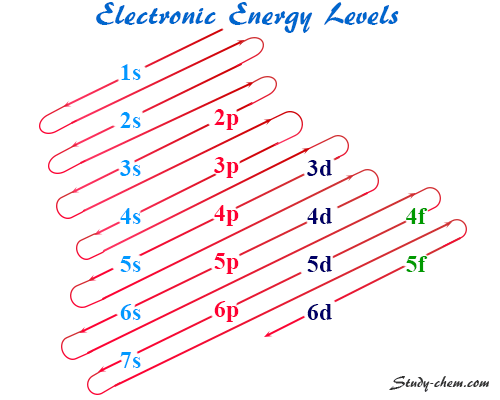Home Atom Electronic Configuration

# Electronic Configuration

Rules, Formula, Examples## Rules for writing electron-configuration

Contents

Electronic configuration or electron configuration of orbitals takes place according to the following rules. The maximum number of electrons in the main quantum shell = 2n2, where n = principal quantum number. Principal quantum number or shell divided into sub shell s, p, d, f. The maximum number of electrons in a subshell = 2(2l +1), where l = 0, 1, 2, 3 for s, p, d, f orbitals respectively. Aufbau building up principle provides the electron filling up process. Hence according to this principle, the orbitals are filled up in the order of increasing energy. Thus orbitals with the lowest energy filled up first while the highest energy orbital filled up in the end.

### Energy Level Diagram

It is difficult for the students to remember the orbital energy level diagram according to the increasing energy level. But a trivial and more convenient diagram was replaced by it for easy to remember.Therefore, the energy levels of orbitals according to this diagram, 1s < 2s < 2p < 3s < 3p < 4s < 3d < 4p < 5s < 4d < 5p < 6s < 4f < 5d < 6p < 7s < 5f…

### Electronic Configuration of s-block Elements

s-block elements consist of one or two-electron or electrons in the s-orbital of the outer quantum shell. Since the common feature generates similar chemical properties among the s-block elements. Therefore, the general electronic configuration of s-block elements is ns1→2.

### 1s-block Chemical Elements

Hydrogen, lithium, sodium, potassium, rubidium, and francium belongs to 1s-block elements in the periodic table with the general electronic configuration of valence electron = ns1.

 Atomic Number Chemical Elements Electron Configuration 1 Hydrogen (H) 1s1 3 Lithium (Li) 1s2 2s1 11 Sodium (Na) [Ne] 3s1 19 Potassium (K) [Ar] 4s1 37 Rubidium (Rb) [Kr] 5s1 55 Cesium (Cs) [Xe] 6s1 87 Francium (Fr) [Rn] 7s1

### 2s-block Chemical Elements

Beryllium, magnesium, calcium, strontium, barium, and radium belongs to 1s-block elements in the periodic table with a general electronic configuration of valence electron = ns2.

 Atomic Number Chemical Elements Electronic Configuration 4 Beryllium (Be) 1s2 2s1 12 Magnesium (Mg) 1s2 2s2 20 Calcium (Ca) [Ne] 3s1 38 Strontium (Sr) [Ar] 4s1 56 Barium (Br) [Kr] 5s1 88 Radium (Ra) [Xe] 6s1